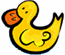Skill 13A Division Introduction: Divide into Groups The first step toward learning division is to make smaller groups from a large group. So, if we are teaching the problem 6 divided by 2, we need for students to ask themselves - How many groups of 2 are in 6? To get the answer they circle each group of two and then count the circles to get the answer. Since there are three circled groups the answer is three. Divide the six ducks into groups of two. Circle each group.